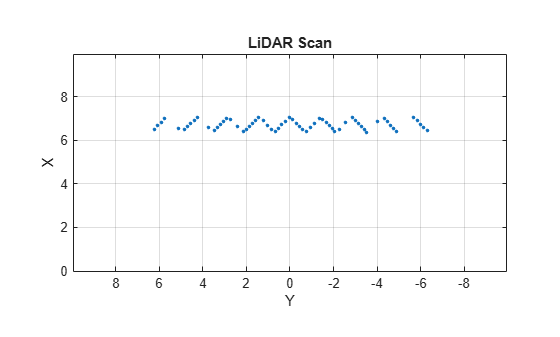rangeSensor

Description

The rangeSensor System object™ is a range-bearing sensor that is capable of outputting range and angle measurements based on the given sensor pose and occupancy map. The range-bearing readings are based on the obstacles in the occupancy map.

To simulate a range-bearing sensor using this object:

1. Create the rangeSensor object and set its properties.

2. Call the object with arguments, as if it were a function.

Creation

Description

example

rbsensor = rangeSensor returns a rangeSensor System object, rbsensor. The sensor is capable of outputting range and angle measurements based on the sensor pose and an occupancy map.

rbsensor = rangeSensor(Name,Value) sets properties for the sensor using one or more name-value pairs. Unspecified properties have default values. Enclose each property name in quotes.

Properties

expand all

Unless otherwise indicated, properties are nontunable, which means you cannot change their values after calling the object. Objects lock when you call them, and the release function unlocks them.

If a property is tunable, you can change its value at any time.

The minimum and maximum detectable range, specified as a 1-by-2 positive real-valued vector. Units are in meters.

Example: [1 15]

Tunable: Yes

Data Types: single | double

Minimum and maximum horizontal detection angle, specified as a 1-by-2 real-valued vector. Units are in radians.

Example: [-pi/3 pi/3]

Data Types: single | double

Resolution of horizontal angle readings, specified as a positive scalar. The resolution defines the angular interval between two consecutive sensor readings. Units are in radians.

Example: 0.01

Data Types: single | double

The standard deviation of range noise, specified as a positive scalar. The range noise is modeled as a zero-mean white noise process with the specified standard deviation. Units are in meters.

Example: 0.01

Tunable: Yes

Data Types: single | double

The standard deviation of horizontal angle noise, specified as a positive scalar. The range noise is modeled as a zero-mean white noise process with the specified standard deviation. Units are in radians.

Example: 0.01

Tunable: Yes

Data Types: single | double

Number of output readings for each pose of the sensor, specified as a positive integer. This property depends on the HorizonalAngle and HorizontalAngleResolution properties.

Data Types: single | double

Usage

Description

example

[ranges,angles] = rbsensor(pose,map) returns the range and angle readings from the 2-D pose information and the ground-truth map.

Input Arguments

expand all

Poses of the sensor in the 2-D map, specified as an N-by-3 real-valued matrix, where N is the number of poses to simulate the sensor. Each row of the matrix corresponds to a pose of the sensor in the order of [x, y, θ]. x and y represent the position of the sensor in the map frame. The units of x and y are in meters. θ is the heading angle of the sensor with respect to the positive x-direction of the map frame. The units of θ are in radians.

Ground-truth map, specified as an occupancyMap or a binaryOccupancyMap object. For the occupancyMap input, the range-bearing sensor considers a cell as occupied and returns a range reading if the occupancy probability of the cell is greater than the value specified by the OccupiedThreshold property of the occupancy map.

Output Arguments

expand all

Range readings, specified as an R-by-N real-valued matrix. N is the number of poses for which the sensor is simulated, and R is the number of sensor readings per pose of the sensor. R is same as the value of the NumReadings property.

Angle readings, specified as an R-by-1 real-valued vector. R is the number of sensor readings per pose of the sensor. R is same as the value of the NumReadings property.

Object Functions

To use an object function, specify the System object as the first input argument. For example, to release system resources of a System object named obj, use this syntax:

release(obj)

expand all

 step Run System object algorithm clone Create duplicate System object

Examples

collapse all

Create a range-bearing sensor.

rbsensor = rangeSensor;

Specify the pose of the sensor and the ground-truth map.

truePose = [0 0 pi/4];
trueMap = binaryOccupancyMap(eye(10));

[ranges, angles] = rbsensor(truePose, trueMap);

Visualize the results using lidarScan.

scan = lidarScan(ranges, angles);
figure
plot(scan)